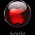### Count 5 Consecutive Zeroes

Query Source   :Excel Macros Google Group
Solution Type   : User Defined Function
Query by        : Lavprasad Kori (MIS Executive, Hindustan UniLever Limited)
Solution by       : Ashish Jain (Microsoft Certified Application Specialist;Lead Trainer, Success Electrons)

Query/Problem :
I want an urgent help for the below metioned data.Attaching hererwith the data file.
I want a help to count the zero value if it comes continues 5 times or more than 5 times then count as 1 time & If it comes continue less than 5 times then ignore it.

Solution:
1. Press Alt + F11.
2. Paste the following code there.(Create a User Defined Function)

Code:

Public Function Count_5_Zeroes() As Integer
On Error Resume Next
Dim Count As Integer
Dim col As Integer
Dim ro As Integer
Do
If WorksheetFunction.Sum(Cells(ro, col).Value, Cells(ro, col - 1).Value, _
Cells(ro, col - 2).Value, Cells(ro, col - 3).Value, Cells(ro, col - 4).Value) = 0 Then
Count = Count + 1
col = col - 4
End If
col = col - 1
Loop Until col < 4
Count_5_Zeroes = Count - 1
On Error GoTo 0
End Function

3. Press Alt+Q.
4. Type "=Count_5_Zeroes()" in AD4 as above in the picture.
5. You're done in just 4 simple steps.Dilip Kumar said…
More dynamic (because its fetch error #VALUE when the cursor is somehere else) Just modified as below:
Public Function Count_5_Zeroes(x As Range) As Integer
On Error Resume Next
Dim Count As Integer
Dim col As Integer
Dim ro As Integer
Application.Volatile

col = Application.WorksheetFunction.CountA(x) + 1
ro = x.Row
Do
If WorksheetFunction.Sum(Cells(ro, col).Value, Cells(ro, col - 1).Value, _
Cells(ro, col - 2).Value, Cells(ro, col - 3).Value, Cells(ro, col - 4).Value) = 0 Then
Count = Count + 1
col = col - 4
End If
col = col - 1
Loop Until col < 4
Count_5_Zeroes = Count - 1
On Error GoTo 0
End FunctionDilip Kumar said…
For only selected range:

Public Function Count_5_Zeroes(x As Range) As Integer
On Error Resume Next
Dim Count As Integer
Dim col As Integer
Dim ro As Integer
Application.Volatile

col = x.coulmn + Application.WorksheetFunction.CountA(x) - 1
ro = x.Row
Do
If WorksheetFunction.Sum(Cells(ro, col).Value, Cells(ro, col - 1).Value, _
Cells(ro, col - 2).Value, Cells(ro, col - 3).Value, Cells(ro, col - 4).Value) = 0 Then
Count = Count + 1
col = col - 4
End If
col = col - 1
Loop Until col < 4
Count_5_Zeroes = Count - 1
On Error GoTo 0
End Function